Courses
Courses for Kids
Free study material
Free LIVE classes
More

# Seven Circle TheoremsLIVE
Join Vedantu’s FREE Mastercalss

## An Introduction to the Seven Circle Theorems

In geometry, we examine several circle theorems related to the various elements of a circle, including a chord, segments, etc. Let's first define a circle before moving on to talk about the circle theorems. A circle is a closed, two-dimensional figure where every point in the plane is equally spaced from a central point. The fixed point and fixed distance are both referred to as the centre and radius, respectively. We will examine many circle theorems used in geometry to address a variety of issues in this article.

## History of the Euclid

Ancient Greek mathematician Euclid was a logician and geometer. The Elements book, often regarded as the "father of geometry," laid the theoretical groundwork for the discipline's development up until the early 19th century.Euclid "Father of Geometry" (300 BCE)

• Name: Euclid

• Born: Around 325 BC

• Died: Around 265 BC

• Field: Mathematics

• Nationality: Greek

## What are the Seven Circle Theorems?

The statements of the seven circle theorems are:

• A chord's angle at its centre is twice as large as its angle at its circumference.

• The diameter at the circumference forms a right angle with the surrounding plane.

• The angles that the same arc subtends at its circumference are equal.

• At the circle's centre, two equal chords subtend two equal angles.

• Two chords are equal if the angles they subtend at the centre are also equal.

• In a cyclic quadrilateral, the opposing angles are supplementary.

• At the point of contact, there is a 90-degree angle between the radius and the tangent.

## Circle Theorems Proofs

Let's prove some of the crucial circle theorems we covered above in this part.

Theorem 1: A chord's angle at its centre is twice as large as its angle at its circumference.

Proof: Take a look at the circle below, where an arc (or segment) AB subtends ∠AOB at the circle's centre O and ∠ACB at a point on its circumference. We must demonstrate that $\angle AOB = 2 \times \angle ACB$. As shown, draw a line segment across points O and C and allow it to once more meet the circle at point D.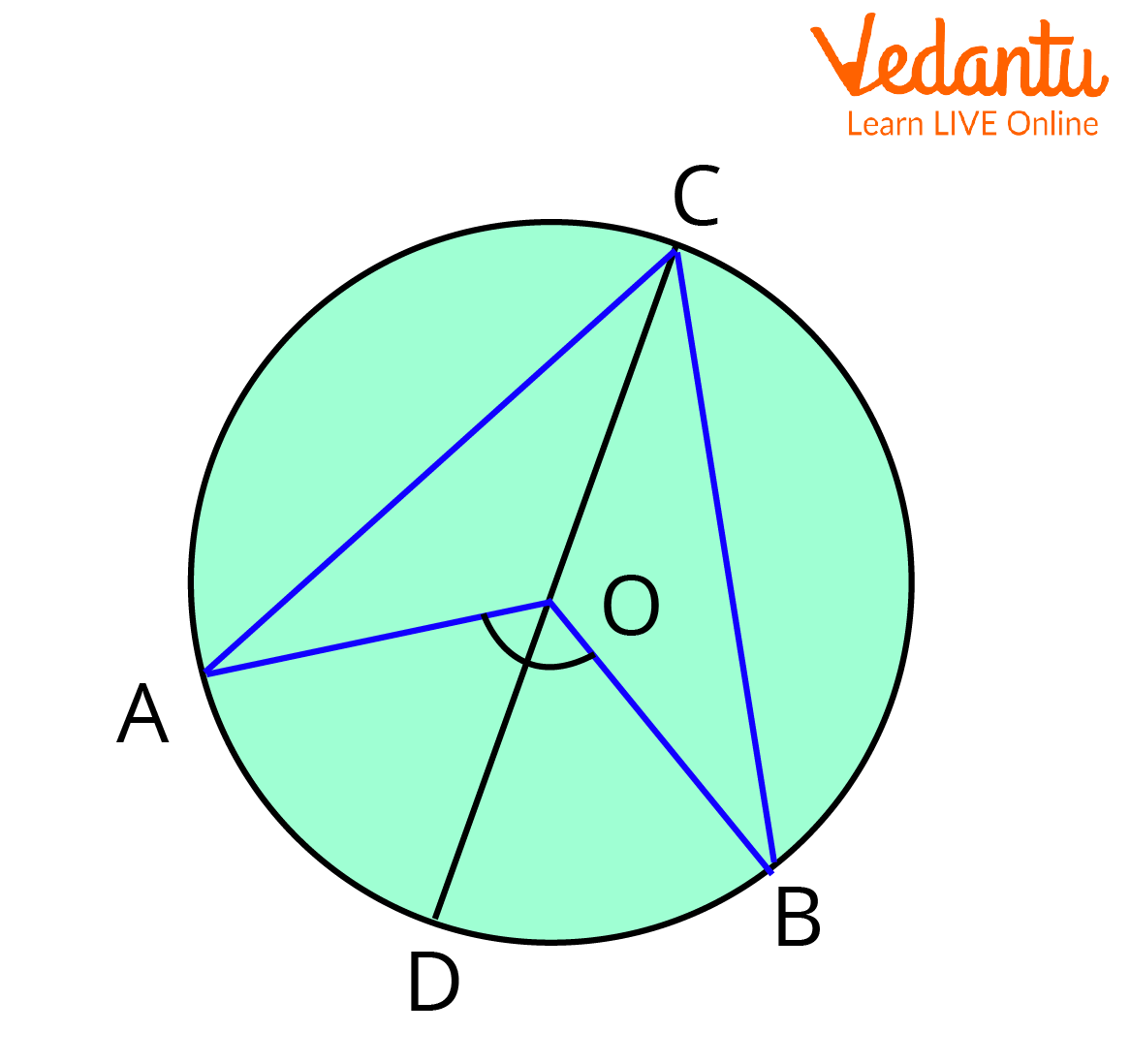Seven Circle Theorem 1

${\bf{\triangle OAC}}$ and ${\bf{\triangle OBC}}$, two triangles, are constructed. As a result, we note the following.

$\angle {\bf{OAC}}{\rm{ }} = {\rm{ }}\angle {\bf{OCA}}$ in ${\bf{\triangle OAC}}$ because ${\bf{OA}}{\rm{ }} = {\rm{ }}{\bf{OC}}$...( radii of the circle )

Because ${\bf{OB}}{\rm{ }} = {\rm{ }}{\bf{OC}}$, in ${\bf{\triangle OBC}}$, $\angle OBC =\angle OCB$ …(radii of the circle)

In triangle COB,

$\angle DOB = \angle OCB + \angle OBC$ (Exterior Angles)…(1)

In triangle COB,

Thus, triangle COB is an isosceles triangle.

Therefore,

$\angle OCB = \angle OCB$ ...(2)

From equations (1) and (2),

$\angle DOB= 2\angle OCB$ …(3)

Similarly,

$\angle AOD = 2\angle ACO$...(4)

Equations (3) and (4) combined:

$\begin{array}{*{20}{l}}{\angle AOD + {\rm{ }}\angle DOB = {\rm{ }}2 \times {\rm{ }}\left( {\angle ACO + {\rm{ }}\angle OCB} \right)}\\{ \Rightarrow {\rm{ }}\angle AOB = {\rm{ }}2 \times {\rm{ }}\angle ACB}\end{array}$

Hence Proved.

Theorem 2: The diameter at the circumference forms a right angle with the surrounding plane.

Proof: Take a look at the illustration below, where AB represents the circle's diameter. We must demonstrate that $\angle ACB = 90^\circ$ .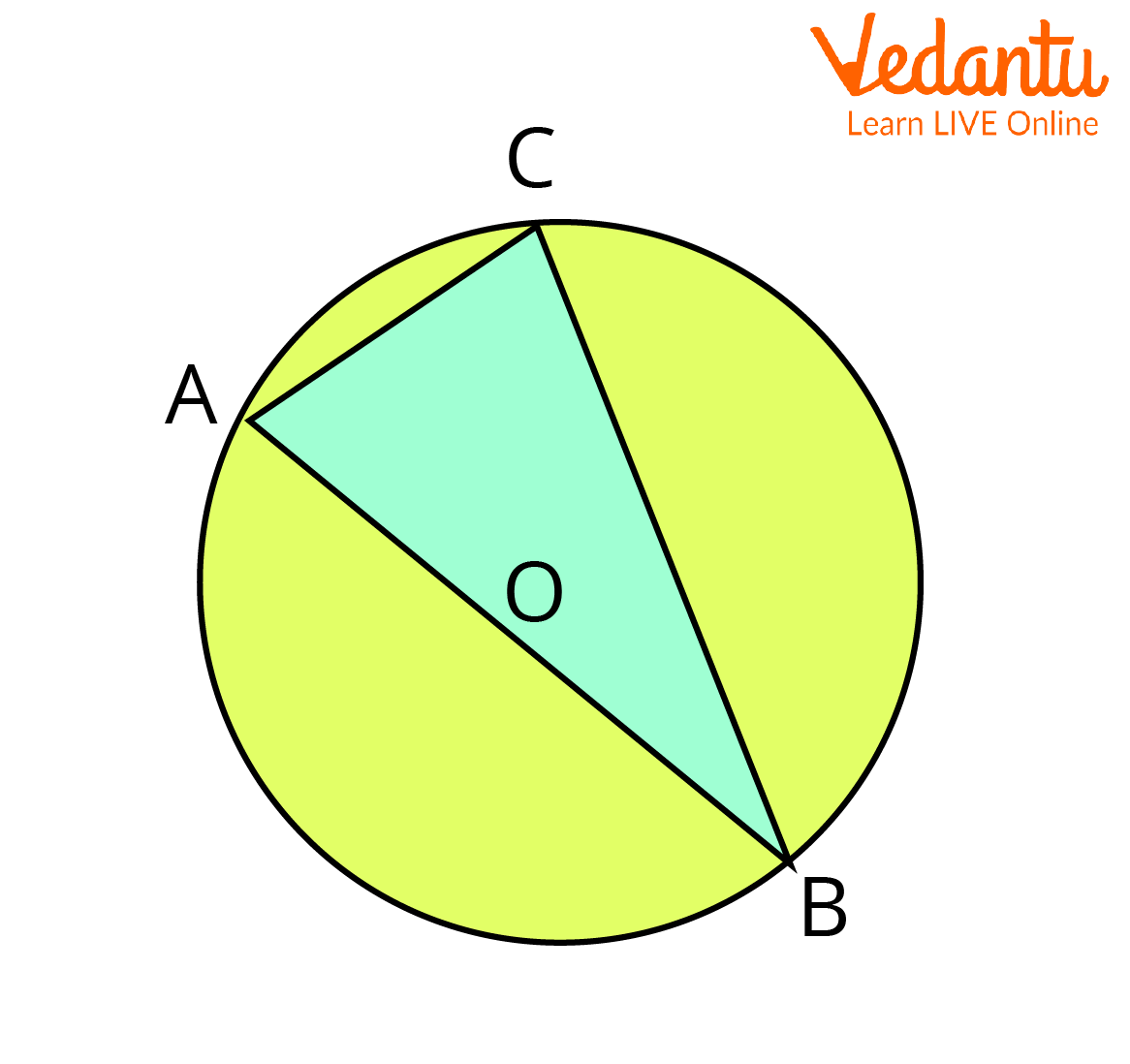Angle in a Semicircle

$\angle {\bf{AOB}} = {\rm{ }}{\bf{2}} \times {\rm{ }}\angle {\bf{ACB}}$ is the result of applying Theorem 1: "The angle subtended by a chord at the centre is twice that subtended by it at the circumference." As AB is a straight line (diameter), $\angle {\bf{AOB}}{\rm{ }} = {\rm{ }}{\bf{180}}^\circ$. Inferring that$\angle {\bf{ACB}}{\rm{ }} = {\rm{ }}{\bf{90}}^\circ ,{\rm{ }}{\bf{2}}{\rm{ }} \times {\rm{ }}\angle {\bf{ACB}}{\rm{ }} = {\rm{ }}{\bf{180}}^\circ$.

Hence Proved.

Theorem 3: The angles that the same arc subtends at its circumference are equal.

Proof: Take a look at the illustration below, which depicts an arc AB subtending angles ACB and ADB at two arbitrary places on the circle, C and D. The circle's centre is O.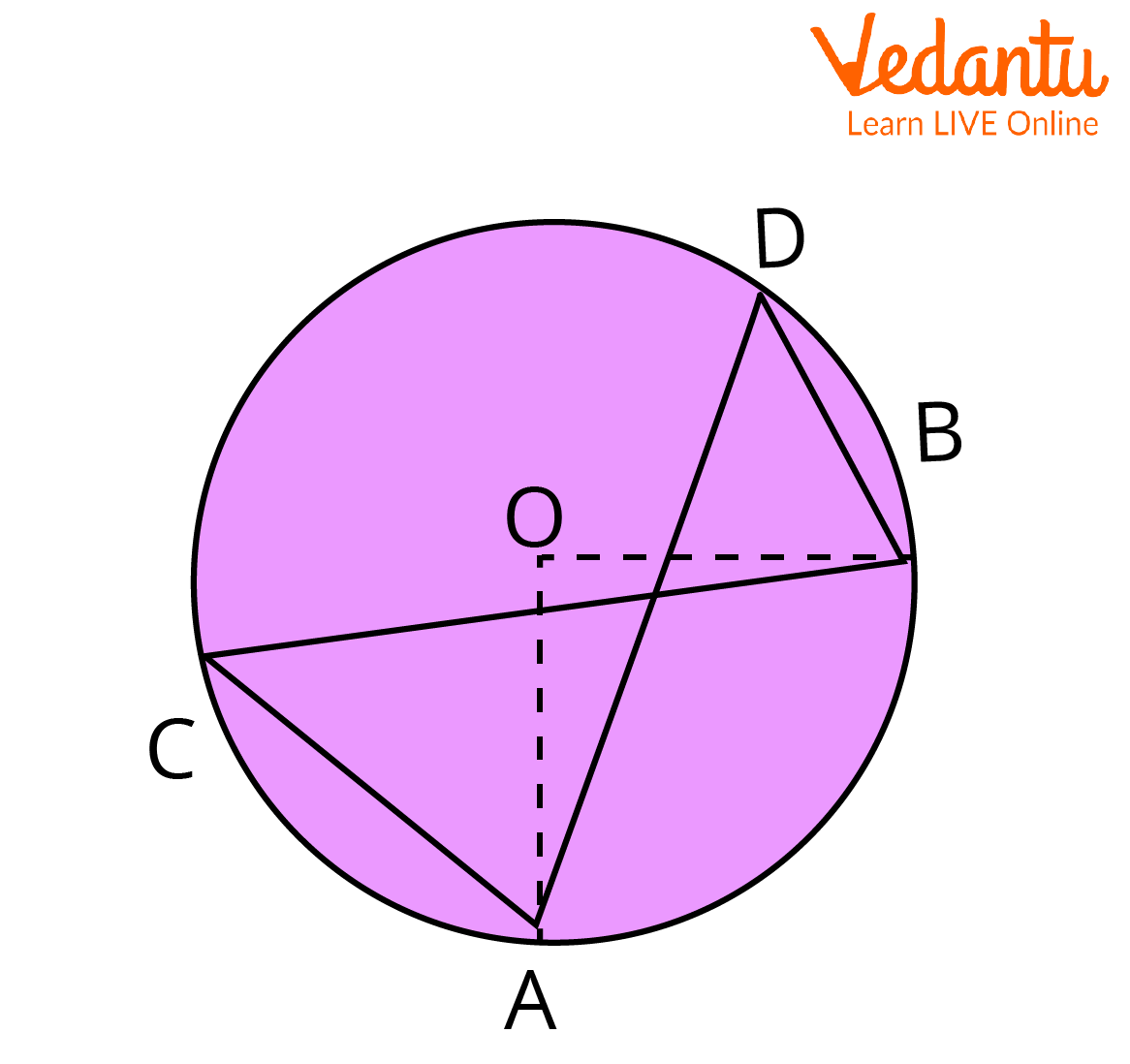Angles in The Same Segment of The Circle

We must demonstrate that $\angle {\bf{ACB}} = {\rm{ }}\angle {\bf{ADB}}$.

The angle a chord subtends at the centre of a circle is twice as large as the angle it subtends at the circumference, according to the circle theorem.

$\begin{array}{*{20}{l}}{\angle ACB = {\rm{ }}1/2 \times {\rm{ }}\angle AOB{\rm{ }} \cdots {\rm{ }}\left( 1 \right)}\\{\angle ADB = {\rm{ }}1/2 \times {\rm{ }}\angle AOB{\rm{ }} \cdots {\rm{ }}\left( 2 \right)}\end{array}$

Equation (1) and equation (2) give us $\angle {\bf{ACB}} = {\rm{ }}\angle {\bf{ADB}}$. Since angles ACB and ADB are arbitrary angles, the conclusion holds for all angles that the same arc subtends.

Hence Proved.

## Solved Examples

1. Consider a circle with the centre O. Utilising circle theorems, determine the value of x.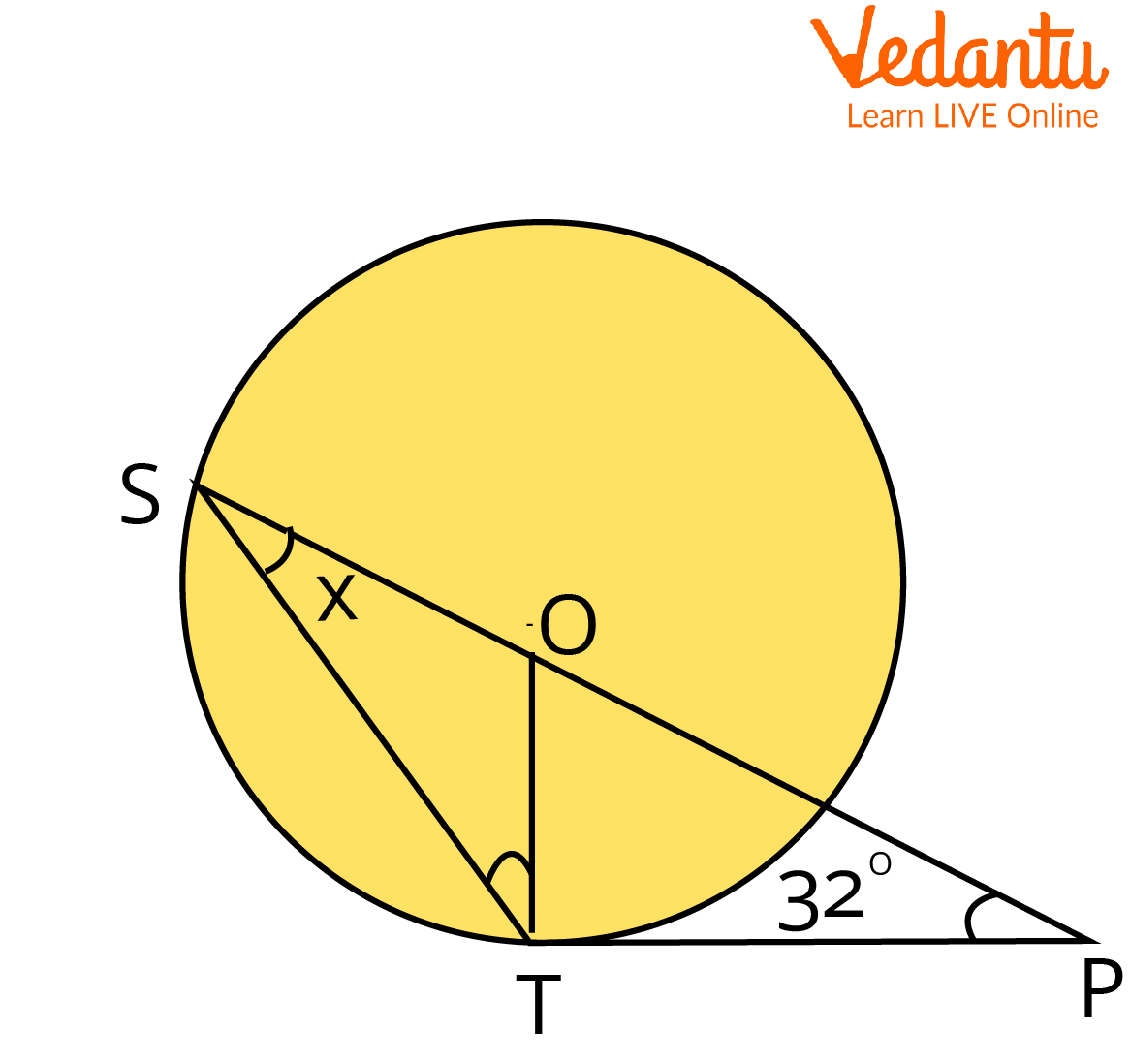A Circle

Ans: A circle with the centre O is supplied, and since the radii OS and OT are equal, OS = OT. OTP = 90° is obtained by using the circle theorem, which states that the angle between the radius and the tangent at the point of contact is 90 degrees. Using the angle sum theorem and the triangle OTP, we have

$\begin{array}{*{20}{l}}{\angle TOP{\rm{ }} + {\rm{ }}\angle OTP{\rm{ }} + {\rm{ }}\angle OPT{\rm{ }} = {\rm{ }}180^\circ }\\{ \Rightarrow {\rm{ }}\angle TOP{\rm{ }} + {\rm{ }}90^\circ {\rm{ }} + {\rm{ }}32^\circ {\rm{ }} = {\rm{ }}180^\circ }\\{ \Rightarrow {\rm{ }}\angle TOP{\rm{ }} = {\rm{ }}180^\circ {\rm{ }} - {\rm{ }}\left( {90^\circ {\rm{ }} + {\rm{ }}32^\circ } \right)}\\{ = {\rm{ }}58^\circ }\end{array}$

Since

$\begin{array}{l}OS{\rm{ }} = {\rm{ }}OT{\rm{ }}\\ \Rightarrow {\rm{ }}\angle OST{\rm{ }} = {\rm{ }}\angle OTS{\rm{ }} = {\rm{ }}x\end{array}$

By the exterior angle theorem,

$\begin{array}{*{20}{l}}{\angle OST{\rm{ }} + {\rm{ }}\angle OTS{\rm{ }} = {\rm{ }}\angle TOP}\\{ \Rightarrow {\rm{ }}x{\rm{ }} + {\rm{ }}x{\rm{ }} = {\rm{ }}58^\circ }\\{ \Rightarrow {\rm{ }}2x{\rm{ }} = {\rm{ }}58^\circ }\\{ \Rightarrow {\rm{ }}x{\rm{ }} = {\rm{ }}29^\circ }\end{array}$

2. Assume the circle with O as the centre is shown below. Using the circle theorems, determine the value of POR.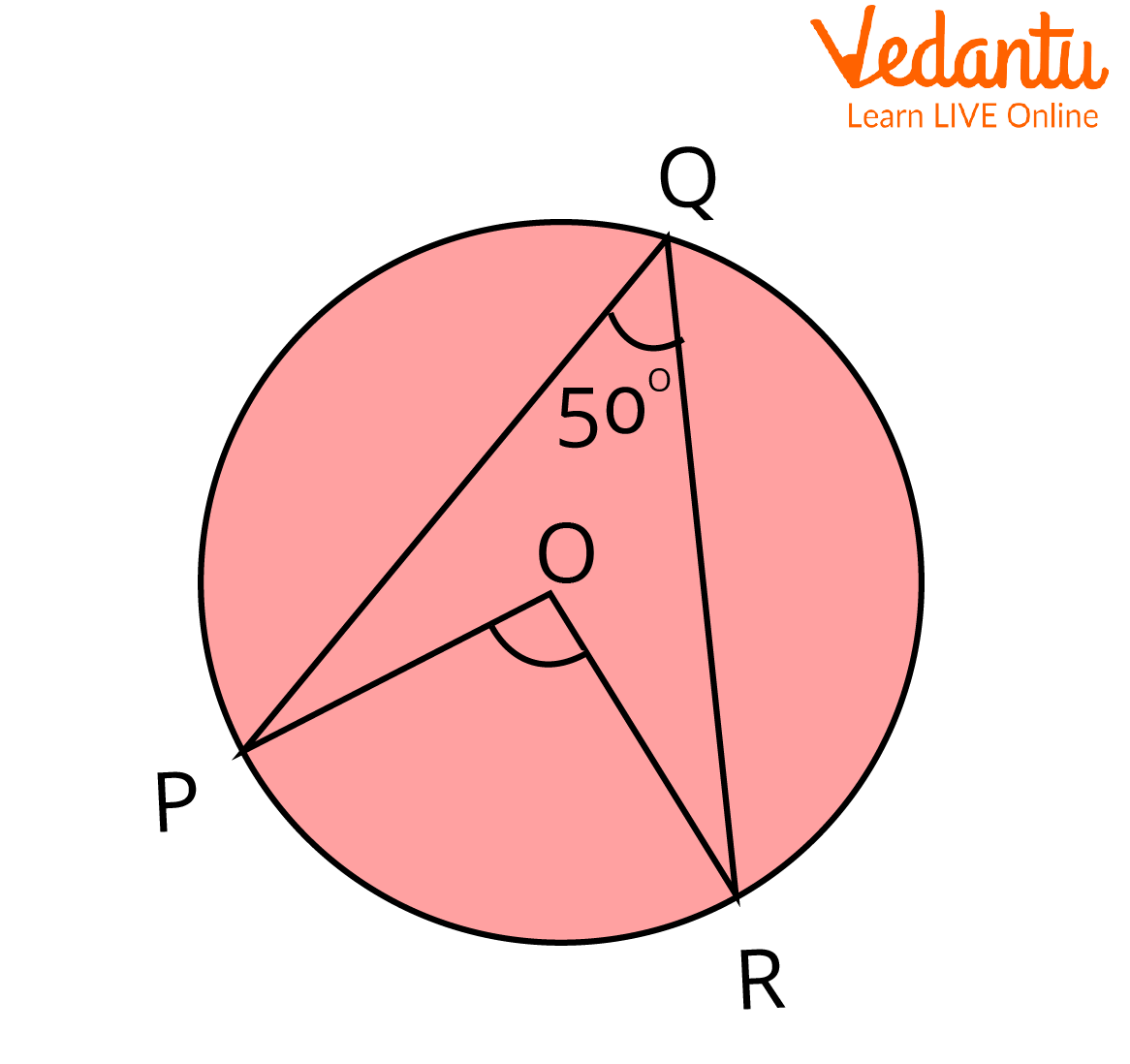A Circle with Centre O

Ans: The circle theorem, which states that a chord's angle at the centre is twice that at the perimeter, will be used to determine the value of angle POR. Thus, $\angle POR{\rm{ }} = {\rm{ }}2\angle PQR$.

Given that $\angle PQR{\rm{ }} = {\rm{ }}50^\circ$, we have

$\begin{array}{*{20}{l}}{\angle POR{\rm{ }} = {\rm{ }}2\angle PQR}\\{ \Rightarrow {\angle POR = {\rm{ }}2{\rm{ }} \times {\rm{ }}50^\circ }}\\{\angle POR= {\rm{ }}100^\circ }\end{array}$

3. Consider the circle with O as the centre is shown below. Using the circle theorems, determine the angle x.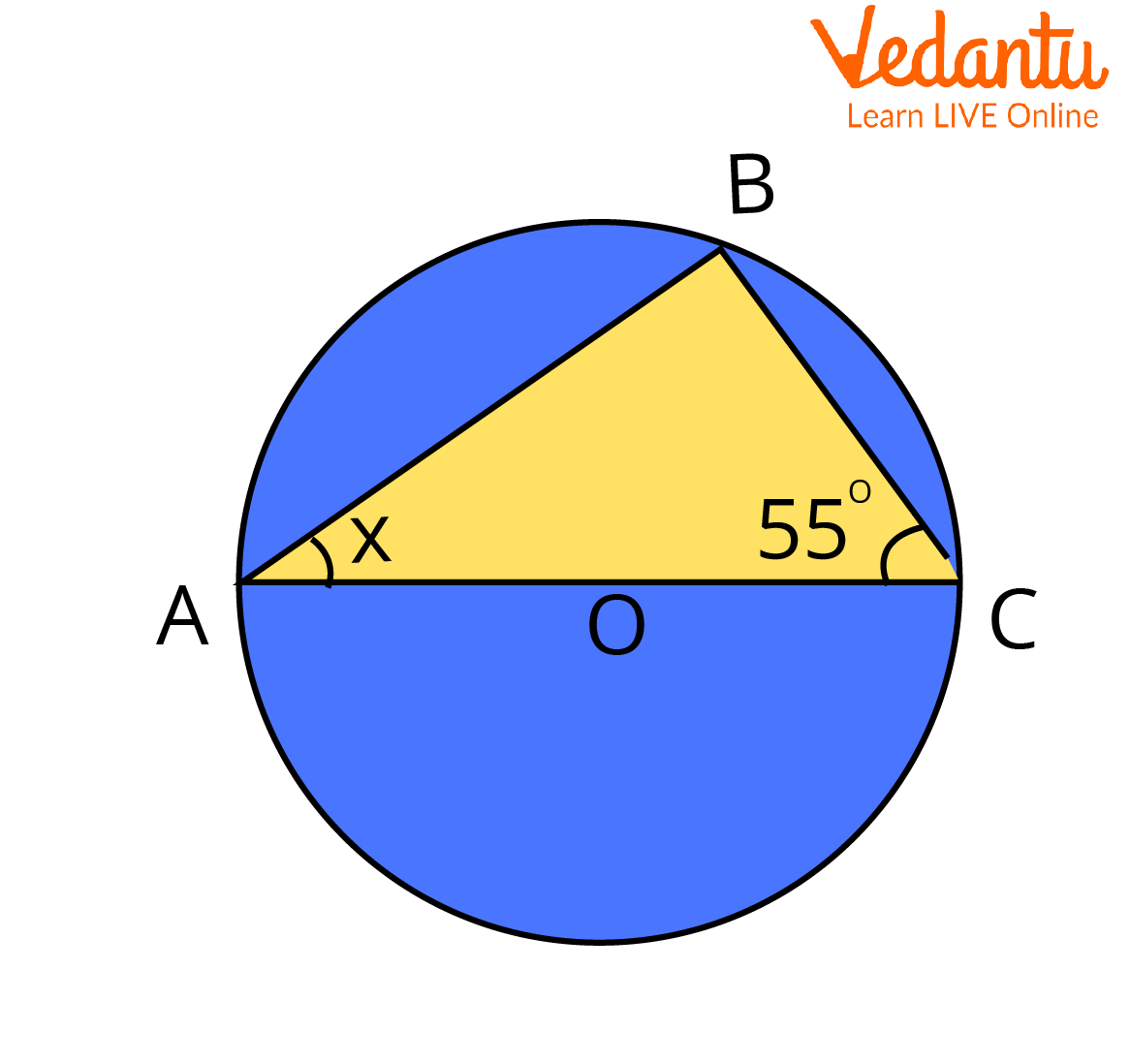Unknown Angle x

Ans: The angle subtended by the diameter at the circumference is a right angle, according to the circle theorem, hence $\angle ABC{\rm{ }} = {\rm{ }}90^\circ$. The triangular sum theorem demonstrates that$\angle BAC{\rm{ }} + {\rm{ }}\angle ACB{\rm{ }} + {\rm{ }}\angle ABC{\rm{ }} = {\rm{ }}180^\circ$.

$\begin{array}{*{20}{l}}{ \Rightarrow {\rm{ }}x{\rm{ }} + {\rm{ }}55^\circ {\rm{ }} + {\rm{ }}90^\circ {\rm{ }} = {\rm{ }}180^\circ }\\{ \Rightarrow {\rm{ }}x{\rm{ }} + {\rm{ }}145^\circ {\rm{ }} = {\rm{ }}180^\circ }\\{ \Rightarrow {\rm{ }}x{\rm{ }} = {\rm{ }}180^\circ {\rm{ }} - {\rm{ }}145^\circ }\\{ = {\rm{ }}35^\circ }\end{array}$

## Applications of the Seven Circle Theorems

Circle theorems are required to address a variety of geometric issues. When we draw angles and lines inside of a circle, we can infer several patterns and theorems that are useful in both theoretical and practical contexts.

## Important Points to Remember

• Geometric theorems about circles are essential conclusions that can be utilised to answer a variety of geometrical problems.

• Angles subtended by the same arc at the circumference are equal.

## Conclusion

The article went into detail about circles. The topic of the seven circle theorems is discussed. Each of the seven has a unique place in Mathematics. Geometrical circle theorems pertain to the several parts of a circle, such as a chord, segments, sector, diameter, tangent, etc.

Last updated date: 02nd Oct 2023
Total views: 104.1k
Views today: 1.04k

## FAQs on Seven Circle Theorems

1. In Circle Theorems, what does subtend mean?

Geometrical assertions known as "circle theorems" outline crucial conclusions about circles. These theorems provide significant information regarding the various parts of a circle, including the chord, segments, sector, diameter, etc. And in circle theorems, "subtend" refers to an angle that is subtended in opposition to an arc or a chord. It is implied when we say that an angle is subtended by an arc that the angle is formed by the lines from the arc on the other side of the circle.

2. What do you understand by SAS Congruence?

Congruent refers to figures that are identical, especially with regard to their size and shape. Congruence describes the relationship between two congruent figures. And to describe the connection between two congruent figures, the phrase SAS congruence, also known as Side Angle Side congruence, is employed. This standard states that two triangles are congruent if their two sides and angle between these two are identical to each other.

3. What do angles mean?

When two lines meet at a point, an angle is created. An "angle" is the measurement of the "opening" between these two rays. It is represented by the symbol ∠. Right angles, acute angles, obtuse angles, straight angles, reflex angles, and complete angles are the six different types of angles. The circularity or rotation of an angle is often measured in degrees and radians. Angles are a common occurrence in daily life. Angles are used by engineers and architects to create highways, structures, and sports venues.